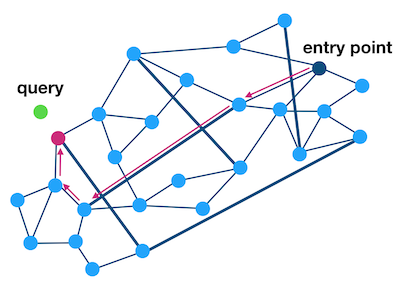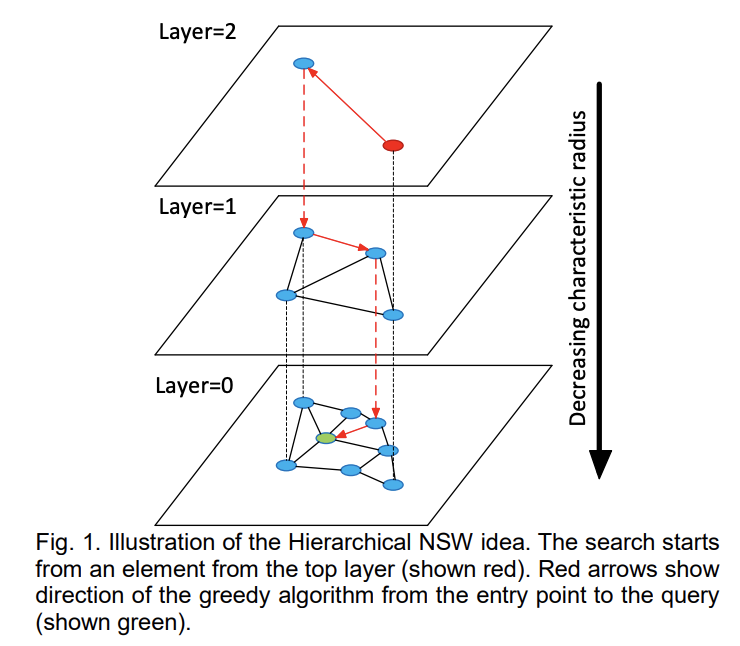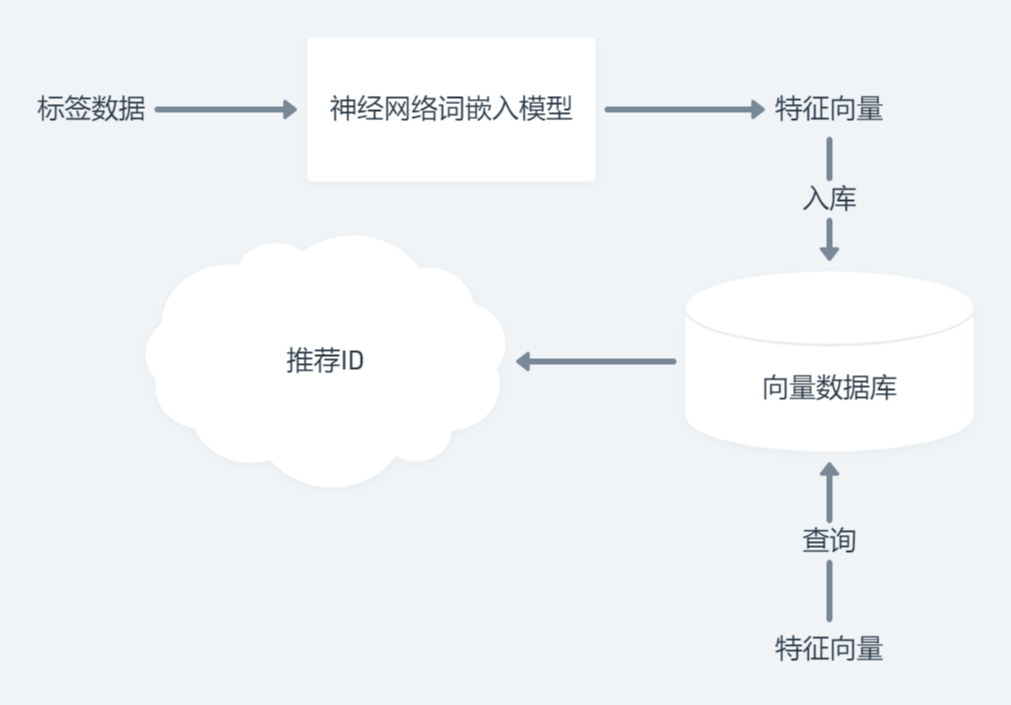# Pixivel 的推荐系统是怎么运作的

Last updated on August 22, 2023 pm

Pixivel 这个项目，大概已经存在了三年甚至四年之久了。这是我唯一一个维护的大型网站（对我来说），也是凝聚了我们众多心血的地方。在此期间，她经历了三次版本大改，无数次的增量迭代。现在的后端和前端系统，我敢说已经十分的健壮，并且已经积累了惊人的用户量。

# 问题分析

1. Pixiv ID；
2. H&W；
3. 作者；
4. 标题；
5. 简介；
6. 标签；
7. 图片链接；

1. 同一个作者画的画可能相关度比较高；
2. 图片自身内容相吻合的画相似度高；
3. 如果一个人看了这张图并点赞，同时他也喜欢看别的图，那么就认为这些图相似度高。；
4. 标签差不多的图相似度高；

1. 需要很牛逼的神经网络模型和数据库才能支撑得起。双塔推荐模型都有点过时了。更别说我这样的小团队运营的站点，自己研发神经网络？不可能。买那么多牛逼的服务器？也不可能。我又不是做淘宝去了，还管什么推荐系统几步曲，什么粗排精排吗？
2. 用户隐私是很重要的问题。有可能你觉得这个没什么，不算隐私。但是对于我来说，任何用户数据的泄露都是很严重的事情，编写隐私政策也是很麻烦的事情（虽然不得不做）都是我写的By ELF。我不能负担得起让用户把行为日志交给我的责任。实际上作为去中心化研究者出身的我们也不愿意让用户妥协自己的隐私，毕竟最理想的还是Zero-Knowledge By ELF

# 算法设计

$$T = {tag_1, tag_2, tag_3, …. , tag_n}$$

$$Jaccard(A, B) = \frac{\left|A\cap B\right|}{\left|A\cup B\right|}$$

## MinHash

1. 有四个集合 $A$, $B$, $C$, $D$
2. 这四个集合都有一些元素，$A = {a, b}$, $B = {c, d, e}$, $C = {a, d, e}$, $D = {a, b, d}$。
3. 它们的并集为$U = {a, b, c, d, e}$。

Element $A$ $B$ $C$ $D$
$a$ 1 0 1 1
$b$ 1 0 0 1
$c$ 0 1 0 0
$d$ 0 1 1 1
$e$ 0 1 1 0

Element $A$ $B$ $C$ $D$
$c$ 0 1 0 0
$a$ 1 0 1 1
$e$ 0 1 1 0
$d$ 0 1 1 1
$b$ 1 0 0 1

### Proof

1. S1 和 S2 的值都为 1
2. S1 和 S2 其中有一个的值为 1
3. S1 和 S2 的值都为 0

$$x = |S1 \cap S2|$$
$$y = |S1 \cup S2|$$

$$Jaccard(S1, S2) = \frac{x}{y}$$

$$P(事件1在第一位) = \frac{x}{y} = Jaccard(S1, S2)$$

Q.E.D.

## LSH (Locality Sensitive Hashing)

MinHash 签名已经能够帮助我们找出相似的集合，那么有没有办法再快一点点？

## 已有算法的缺点

MinHash 和 LSH 归根结底依靠的是概率，所以它们的精确率（以及召回率）不是很高。至于实现的速度和空间利用，也没有达到我比较满意的程度。

1. 欧几里得度量 (Euclidean Distance)
对于 n 维空间的两点 p, q 来说，距离d：
$$d(p,q)={\sqrt {(p_{1}-q_{1})^{2}+(p_{2}-q_{2})^{2}+\cdots +(p_{i}-q_{i})^{2}+\cdots +(p_{n}-q_{n})^{2}}}$$

2. 余弦相似度 (Cosine similarity)
顾名思义，就是测量两个向量之间的夹角：
$${\text{Cosine Similarity}}=S_{C} (A,B):=\cos(\theta)={\mathbf{A} \cdot \mathbf{B} \over|\mathbf{A} ||\mathbf{B} |}={\frac{\sum\limits_{i=1}^{n}{A_{i}B_{i}}}{ {\sqrt{\sum\limits_{i=1}^{n}{A_{i}^{2}}}}{\sqrt{\sum\limits_{i=1}^{n}{B_{i}^{2}}}}}}$$

### HNSW（Hierarchical Navigable Small World）索引

1. 不会出现孤点没有任何连接，导致无法被搜索到；
2. 相似的点互相连接，保证准确性；
3. 连接数量最少，提高效率；### Word Embedding

1. 两个即使表现不同但是语义相似的词，可以被临近匹配。
2. 多个词在集合里，如果我们想要取得整个集合的特征，直接计算每个词的特征，然后再经过向量的平均就可以了。## 神经网络+最邻近搜索Over.

## 实践效果## 结尾

Pixivel 的推荐系统是怎么运作的
http://elfile4138.moe/2022/07/Pixivel-的推荐系统是怎么运作的/
Author
Rorical
Posted on
July 24, 2022
Updated on
August 22, 2023